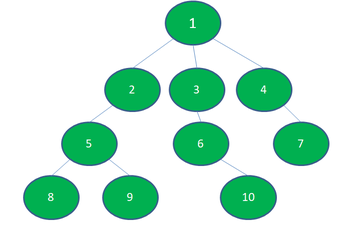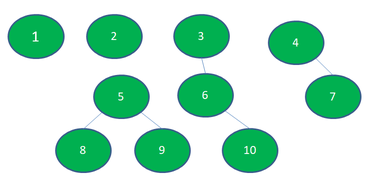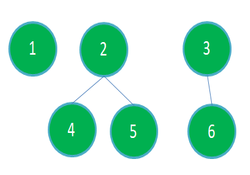GeeksforGeeks App
Open AppBrowser
Continue

# Minimum number of Nodes to be removed such that no subtree has more than K nodes

Given a tree with N nodes value from 1 to N and (N – 1) Edges and a number K, the task is to remove the minimum number of nodes from the tree such that every subtree will have at most K nodes. Removing Nodes will remove the edges from that nodes to all the other connected nodes.

Examples:

Input: N = 10, K = 3, Below is the graph:Output:
Number of nodes removed: 2
Removed Nodes: 2 1
Explanation:
After removing the nodes 1 and 2, here, no subtree or tree has more than 3 nodes. Below is the resulting graph:Input: N = 6, K = 3, Below is the graph:Output:
Number of nodes removed: 1
Removed Nodes: 1
Explanation:
After removing the nodes 1, here, no subtree or tree has more than 3 nodes. Below is the resulting graph:Approach: The idea is to observe that the number of nodes in the subtree of a node X is the sum of the number of nodes in the subtree of its children and the node itself. Below are the steps:

• Use Dynamic Programming and DFS to store the count of nodes in the subtree of each node easily.
• Now, to have no node with subtree having more than K nodes, the idea is to remove the node whenever it has more than K nodes in its subtree, and pass 0 to its parent.
• In the above step, we are having each node with nodes in its subtree not greater than K and minimizing the number of node removals.

Below is the implementation of the above approach:

## C++

 `// C++ program for the above approach``#include ``using` `namespace` `std;` `#define N 20` `// Function to perform DFS Traversal``int` `dfs(vector<``bool``> &visited, ``int` `s,``        ``int` `&K, ``int` `&removals,``        ``vector<``int``> &removed_nodes,``        ``vector> &adj)``{``  ` `  ``// Mark the node as true``  ``visited[s] = ``true``;``  ``int` `nodes = 1;` `  ``// Traverse adjacency list``  ``// of child node``  ``for``(``int` `child : adj[s])``  ``{``    ` `    ``// If already visited then``    ``// omit the node``    ``if` `(visited[child])``      ``continue``;` `    ``// Add number of nodes``    ``// in subtree``    ``nodes += dfs(visited, child, K,``                 ``removals, removed_nodes,``                 ``adj);``  ``}` `  ``if` `(nodes > K)``  ``{``    ` `    ``// Increment the count``    ``removals++;``    ``removed_nodes.push_back(s);``    ``nodes = 0;``  ``}` `  ``// Return the nodes``  ``return` `nodes;``}` `// Function to add edges in graph``void` `addEdge(vector> &adj,``             ``int` `a, ``int` `b)``{``  ``adj[a].push_back(b);``  ``adj[b].push_back(a);``}` `// Function that finds the number``// of nodes to be removed such that``// every subtree has size at most K``void` `findRemovedNodes(vector<``bool``> &visited, ``int` `K,``                      ``int` `&removals,``                      ``vector<``int``> &removed_nodes,``                      ``vector> &adj)``{``  ` `  ``// Function Call to find the``  ``// number of nodes to remove``  ``dfs(visited, 1, K, removals,``      ``removed_nodes, adj);` `  ``// Print Removed Nodes``  ``cout << ``"Number of nodes removed: "``       ``<< removals << endl;` `  ``cout << ``"Removed Nodes: "``;``  ``for``(``int` `node : removed_nodes)``  ``{``    ``cout << node << ``" "``;``  ``}``}` `// Driver Code``int` `main()``{``  ` `  ``// Variables used to store data globally``  ``vector<``bool``> visited(N);``  ``int` `K;``  ``int` `removals = 0;``  ``vector<``int``> removed_nodes;` `  ``// Adjacency list representation of tree``  ``vector> adj(N);` `  ``// Insert of nodes in graph``  ``addEdge(adj, 1, 2);``  ``addEdge(adj, 1, 3);``  ``addEdge(adj, 2, 4);``  ``addEdge(adj, 2, 5);``  ``addEdge(adj, 3, 6);` `  ``// Required subtree size``  ``K = 3;``  ` `  ``// Function Call``  ``findRemovedNodes(visited, K, removals,``                   ``removed_nodes, adj);` `  ``return` `0;``}` `// This code is contributed by sanjeev2552`

## Java

 `// Java program for the above approach``import` `java.util.*;` `class` `GFG {` `    ``// Variables used to store data globally``    ``static` `final` `int` `N = ``20``;``    ``static` `boolean` `visited[] = ``new` `boolean``[N];``    ``static` `int` `K;``    ``static` `int` `removals = ``0``;``    ``static` `ArrayList removed_nodes``        ``= ``new` `ArrayList<>();` `    ``// Adjacency list representation of tree``    ``static` `ArrayList > adj``        ``= ``new` `ArrayList<>();` `    ``// Function to perform DFS Traversal``    ``static` `int` `dfs(``int` `s)``    ``{``        ``// Mark the node as true``        ``visited[s] = ``true``;``        ``int` `nodes = ``1``;` `        ``// Traverse adjacency list``        ``// of child node``        ``for` `(Integer child : adj.get(s)) {` `            ``// If already visited then``            ``// omit the node``            ``if` `(visited[child])``                ``continue``;` `            ``// Add number of nodes``            ``// in subtree``            ``nodes += dfs(child);``        ``}` `        ``if` `(nodes > K) {` `            ``// Increment the count``            ``removals++;``            ``removed_nodes.add(s);``            ``nodes = ``0``;``        ``}` `        ``// Return the nodes``        ``return` `nodes;``    ``}` `    ``// Function to add edges in graph``    ``static` `void` `addEdge(``int` `a, ``int` `b)``    ``{``        ``adj.get(a).add(b);``        ``adj.get(b).add(a);``    ``}` `    ``// Function that finds the number``    ``// of nodes to be removed such that``    ``// every subtree has size at most K``    ``public` `static` `void` `findRemovedNodes(``int` `K)``    ``{``        ``// Function Call to find the``        ``// number of nodes to remove``        ``dfs(``1``);` `        ``// Print Removed Nodes``        ``System.out.println(``"Number of nodes"``                           ``+ ``" removed: "``                           ``+ removals);` `        ``System.out.print(``"Removed Nodes: "``);``        ``for` `(``int` `node : removed_nodes)``            ``System.out.print(node + ``" "``);``    ``}` `    ``// Driver Code``    ``public` `static` `void` `main(String[] args)``    ``{``        ``// Creating list for all nodes``        ``for` `(``int` `i = ``0``; i < N; i++)``            ``adj.add(``new` `ArrayList<>());` `        ``// Insert of nodes in graph``        ``addEdge(``1``, ``2``);``        ``addEdge(``1``, ``3``);``        ``addEdge(``2``, ``4``);``        ``addEdge(``2``, ``5``);``        ``addEdge(``3``, ``6``);` `        ``// Required subtree size``        ``K = ``3``;` `        ``// Function Call``        ``findRemovedNodes(K);``    ``}``}`

## Python3

 `# Python3 program for the above approach` `# Variables used to store data globally``N ``=` `20``visited ``=` `[``False` `for` `i ``in` `range``(N)]``K ``=` `0``removals ``=` `0``removed_nodes ``=` `[]` `# Adjacency list representation of tree``adj ``=` `[[] ``for` `i ``in` `range``(N)]` `# Function to perform DFS Traversal``def` `dfs(s):``    ` `    ``global` `removals``    ` `    ``# Mark the node as true``    ``visited[s] ``=` `True``    ``nodes ``=` `1` `    ``# Traverse adjacency list``    ``# of child node``    ``for` `child ``in` `adj[s]:` `        ``# If already visited then``        ``# omit the node``        ``if` `(visited[child]):``            ``continue` `        ``# Add number of nodes``        ``# in subtree``        ``nodes ``+``=` `dfs(child)` `    ``if` `(nodes > K):` `        ``# Increment the count``        ``removals ``+``=` `1``        ``removed_nodes.append(s)``        ``nodes ``=` `0` `    ``# Return the nodes``    ``return` `nodes` `# Function to add edges in graph``def` `addEdge(a, b):` `    ``adj[a].append(b)``    ``adj[b].append(a)` `# Function that finds the number``# of nodes to be removed such that``# every subtree has size at most K``def` `findRemovedNodes(K):` `    ``# Function Call to find the``    ``# number of nodes to remove``    ``dfs(``1``)` `    ``# Print Removed Nodes``    ``print``(``"Number of nodes removed: "``, removals)` `    ``print``(``"Removed Nodes: "``, end ``=` `' '``)``    ` `    ``for` `node ``in` `removed_nodes:``        ``print``(node, end ``=` `' '``)``    ` `# Driver Code``if` `__name__ ``=``=` `"__main__"``:` `    ``# Insert of nodes in graph``    ``addEdge(``1``, ``2``)``    ``addEdge(``1``, ``3``)``    ``addEdge(``2``, ``4``)``    ``addEdge(``2``, ``5``)``    ``addEdge(``3``, ``6``)` `    ``# Required subtree size``    ``K ``=` `3` `    ``# Function Call``    ``findRemovedNodes(K)` `# This code is contributed by rutvik_56`

## C#

 `// C# program for the above approach``using` `System;``using` `System.Collections.Generic;` `class` `GFG{` `  ``// Variables used to store data globally``  ``static` `readonly` `int` `N = 20;``  ``static` `bool` `[]visited = ``new` `bool``[N];``  ``static` `int` `K;``  ``static` `int` `removals = 0;``  ``static` `List<``int``> removed_nodes``    ``= ``new` `List<``int``>();` `  ``// Adjacency list representation of tree``  ``static` `List > adj``    ``= ``new` `List>();` `  ``// Function to perform DFS Traversal``  ``static` `int` `dfs(``int` `s)``  ``{``    ``// Mark the node as true``    ``visited[s] = ``true``;``    ``int` `nodes = 1;` `    ``// Traverse adjacency list``    ``// of child node``    ``foreach` `(``int` `child ``in` `adj[s])``    ``{` `      ``// If already visited then``      ``// omit the node``      ``if` `(visited[child])``        ``continue``;` `      ``// Add number of nodes``      ``// in subtree``      ``nodes += dfs(child);``    ``}` `    ``if` `(nodes > K)``    ``{` `      ``// Increment the count``      ``removals++;``      ``removed_nodes.Add(s);``      ``nodes = 0;``    ``}` `    ``// Return the nodes``    ``return` `nodes;``  ``}` `  ``// Function to add edges in graph``  ``static` `void` `addEdge(``int` `a, ``int` `b)``  ``{``    ``adj[a].Add(b);``    ``adj[b].Add(a);``  ``}` `  ``// Function that finds the number``  ``// of nodes to be removed such that``  ``// every subtree has size at most K``  ``public` `static` `void` `findRemovedNodes(``int` `K)``  ``{``    ``// Function Call to find the``    ``// number of nodes to remove``    ``dfs(1);` `    ``// Print Removed Nodes``    ``Console.WriteLine(``"Number of nodes"` `+    ``                           ``" removed: "` `+``                               ``removals);` `    ``Console.Write(``"Removed Nodes: "``);``    ``foreach` `(``int` `node ``in` `removed_nodes)``      ``Console.Write(node + ``" "``);``  ``}` `  ``// Driver Code``  ``public` `static` `void` `Main(String[] args)``  ``{``    ``// Creating list for all nodes``    ``for` `(``int` `i = 0; i < N; i++)``      ``adj.Add(``new` `List<``int``>());` `    ``// Insert of nodes in graph``    ``addEdge(1, 2);``    ``addEdge(1, 3);``    ``addEdge(2, 4);``    ``addEdge(2, 5);``    ``addEdge(3, 6);` `    ``// Required subtree size``    ``K = 3;` `    ``// Function Call``    ``findRemovedNodes(K);``  ``}``}` `// This code is contributed by Rohit_ranjan`

## Javascript

 ``

Output

```Number of nodes removed: 1
Removed Nodes: 1```

Time Complexity: O(N)
Auxiliary Space: O(N)

My Personal Notes arrow_drop_up